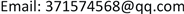﻿ 单调保k变换半群ORn(K)的Green关系 Green’s Relations of Semigroup Monotone and k-Preserving Transformations

Pure Mathematics
Vol. 09  No. 02 ( 2019 ), Article ID: 29476 , 6 pages
10.12677/PM.2019.92030

Green’s Relations of Semigroup Monotone and k-Preserving Transformations

Yan Sun

School of Mathematics, Guizhou Normal University, Guiyang GuizhouReceived: Mar. 6th, 2019; accepted: Mar. 20th, 2019; published: Mar. 27th, 2019ABSTRACT

Let ${X}_{n}=\left\{1,2,\cdots ,n\right\}$ denote a finite set, $Sin{g}_{n}$ is Singular transformation semigroups on it. ${O}_{n}$ and ${R}_{n}$ is Order-preserving and anti-order-preserving transformation semigroups of $Sin{g}_{n}$ . Let $O{R}_{n}\left(k\right)=\left\{\alpha \in {O}_{n}:k\alpha =k\right\}\cup \left\{\alpha \in {R}_{n}:k\alpha =k\right\}$ , this paper describes Green’s relations of $O{R}_{n}\left(k\right)$ .

Keywords:Order Preserving, Transformation Semigroups, Green’s Relations${X}_{n}=\left\{1,2,\cdots ,n\right\}$ 是有限集， $Sin{g}_{n}$${X}_{n}$ 上的奇异变换半群， ${O}_{n}$${R}_{n}$$Sin{g}_{n}$ 上的保序变换之集和反保序变换之集，令 $O{R}_{n}\left(k\right)=\left\{\alpha \in {O}_{n}:k\alpha =k\right\}\cup \left\{\alpha \in {R}_{n}:k\alpha =k\right\}$ ，刻画了半群 $O{R}_{n}\left(k\right)$ 的Green关系。1. 引言与准备

${X}_{n}=\left\{1,2,\cdots ,n\right\}$ 并赋予自然序， ${T}_{n}$${X}_{n}$ 上的全变换半群， $Sin{g}_{n}=\left\{\alpha \in {T}_{n}:|im\alpha |${X}_{n}$ 上的奇异变换半群。设 $\alpha \in Sin{g}_{n}$ ，若对任意 $x,y\in {X}_{n}$ ，有 $x\le y⇒x\alpha \le y\alpha \left(x\le y⇒x\alpha \ge y\alpha \right)$ ，称 $\alpha$ 是保序(反序)的。设 ${O}_{n}$${R}_{n}$ 分别为 $Sin{g}_{n}$ 上的保序变换之集和反保序变换之集，则 ${O}_{n}$$Sin{g}_{n}$ 的子半群，称 ${O}_{n}$$Sin{g}_{n}$ 上的保序变换半群。对任意 1 ≤ k ≤ n，令

${O}_{n}\left(k\right)=\left\{\alpha \in {O}_{n}:k\alpha =k\right\}$

${R}_{n}\left(k\right)=\left\{\alpha \in {R}_{n}:k\alpha =k\right\}$

${O}_{n}\left(k\right)$${R}_{n}\left(k\right)$$Sin{g}_{n}$ 的子集。

$O{R}_{n}\left(k\right)={O}_{n}\left(k\right)\cup {R}_{n}\left(k\right)$

$L=\left\{\left(a,b\right):a,b\in S,{S}^{1}a={S}^{1}b\right\}$

$R=\left\{\left(a,b\right):a,b\in S,a{S}^{1}=b{S}^{1}\right\}$

$D=L\cdot R$

$H=L\cap R$

$J=\left\{\left(a,b\right):a,b\in S,{S}^{1}a{S}^{1}={S}^{1}b{S}^{1}\right\}$

2. 主要结果及证明

${A}_{i},{A}_{j}\subseteq {X}_{n}$ ，对任意 $x\in {A}_{i},y\in {A}_{j}$ ，都有 $x ，记作 ${A}_{i}<{A}_{j}$

$1\le k\le n$$\alpha \in O{R}_{n}\left(k\right)$ ，记：

${p}_{\alpha }\left(k\right)=\left\{y\in im\left(\alpha \right):y

${Q}_{\alpha }\left(k\right)=\left\{y\in im\left(\alpha \right):y

$\left(\begin{array}{c}{A}_{i}\\ \\ {a}_{i}\end{array}|\begin{array}{c}\\ {a}_{i}\in {P}_{\alpha }\left(k\right)\\ i=1,2,\cdots ,{p}_{\alpha }\left(k\right)\end{array}\begin{array}{c}{K}_{\alpha }\\ \\ k\end{array}\begin{array}{c}{B}_{j}\\ \\ {b}_{j}\end{array}|\begin{array}{c}\\ {b}_{j}\in {Q}_{\alpha }\left(k\right)\\ j=1,2,\cdots ,{q}_{\alpha }\left(k\right)\right)\end{array}$

$\left(\begin{array}{c}{A}_{i}\\ \\ {a}_{i}\end{array}|\begin{array}{c}\\ {a}_{i}\in {Q}_{\alpha }\left(k\right)\\ i=1,2,\cdots ,{q}_{\alpha }\left(k\right)\end{array}\begin{array}{c}{K}_{\alpha }\\ \\ k\end{array}\begin{array}{c}{B}_{j}\\ \\ {b}_{j}\end{array}|\begin{array}{c}\\ {b}_{j}\in {P}_{\alpha }\left(k\right)\\ j=1,2,\cdots ,{p}_{\alpha }\left(k\right)\right)\end{array}$

$x\delta =\left\{\begin{array}{l}\mathrm{min}\left(x\alpha \right){\beta }^{-1},\text{\hspace{0.17em}}\text{\hspace{0.17em}}x\in {X}_{n}\\left\{k\right\},\\ k,\text{\hspace{0.17em}}\text{\hspace{0.17em}}\text{\hspace{0.17em}}\text{\hspace{0.17em}}\text{\hspace{0.17em}}\text{\hspace{0.17em}}\text{\hspace{0.17em}}\text{\hspace{0.17em}}\text{\hspace{0.17em}}\text{\hspace{0.17em}}\text{\hspace{0.17em}}\text{\hspace{0.17em}}\text{\hspace{0.17em}}\text{\hspace{0.17em}}\text{\hspace{0.17em}}\text{\hspace{0.17em}}\text{\hspace{0.17em}}\text{ }\text{ }x=k;\end{array}$

$x\gamma =\left\{\begin{array}{l}\mathrm{min}\left(x\beta \right){\alpha }^{-1},\text{\hspace{0.17em}}\text{\hspace{0.17em}}x\in {X}_{n}\\left\{k\right\},\\ k,\text{\hspace{0.17em}}\text{\hspace{0.17em}}\text{\hspace{0.17em}}\text{\hspace{0.17em}}\text{\hspace{0.17em}}\text{\hspace{0.17em}}\text{\hspace{0.17em}}\text{\hspace{0.17em}}\text{\hspace{0.17em}}\text{\hspace{0.17em}}\text{\hspace{0.17em}}\text{\hspace{0.17em}}\text{\hspace{0.17em}}\text{\hspace{0.17em}}\text{\hspace{0.17em}}\text{\hspace{0.17em}}\text{ }\text{ }\text{\hspace{0.17em}}x=k;\end{array}$

$\alpha =\left(\begin{array}{ccccccccc}{A}_{1}& {A}_{2}& \cdots & {A}_{l}& {K}_{\alpha }& {B}_{1}& {B}_{2}& \cdots & {B}_{m}\\ {a}_{1}& {a}_{2}& \cdots & {a}_{l}& k& {b}_{1}& {b}_{2}& \cdots & {b}_{m}\end{array}\right)$

$\beta =\left(\begin{array}{ccccccccc}{A}_{1}& {A}_{2}& \cdots & {A}_{l}& {K}_{\alpha }& {B}_{1}& {B}_{2}& \cdots & {B}_{m}\\ {\stackrel{˜}{a}}_{1}& {\stackrel{˜}{a}}_{2}& \cdots & {\stackrel{˜}{a}}_{l}& k& {\stackrel{˜}{b}}_{1}& {\stackrel{˜}{b}}_{2}& \cdots & {\stackrel{˜}{b}}_{m}\end{array}\right)$

$\delta =\left(\begin{array}{ccccccc}\left[1,{\stackrel{˜}{a}}_{1}\right]& \left({\stackrel{˜}{a}}_{1},{\stackrel{˜}{a}}_{2}\right]& \cdots & \left({\stackrel{˜}{a}}_{l},k\right]& \left(k,{\stackrel{˜}{b}}_{1}\right]& \cdots & \left({\stackrel{˜}{b}}_{m-1},n\right]\\ {a}_{1}& {a}_{2}& \cdots & k& {b}_{1}& \cdots & {b}_{m}\end{array}\right)$

$\gamma =\left(\begin{array}{ccccccc}\left[1,{a}_{1}\right]& \left({a}_{1},{a}_{2}\right]& \cdots & \left({a}_{l},k\right]& \left(k,{\stackrel{˜}{b}}_{1}\right]& \cdots & \left({b}_{m-1},n\right]\\ {\stackrel{˜}{a}}_{1}& {\stackrel{˜}{a}}_{2}& \cdots & k& {b}_{1}& \cdots & {\stackrel{˜}{b}}_{m}\end{array}\right)$

$\delta =\left(\begin{array}{cccccc}\left[1,{\stackrel{˜}{b}}_{m}\right]& \cdots & \left({\stackrel{˜}{b}}_{1},k\right]& \left(k,{\stackrel{˜}{a}}_{l}\right]& \cdots & \left({\stackrel{˜}{a}}_{2},n\right]\\ {b}_{m}& \cdots & k& {a}_{l}& \cdots & {a}_{1}\end{array}\right)$

$\gamma =\left(\begin{array}{cccccc}\left[1,{b}_{m}\right]& \cdots & \left({b}_{1},k\right]& \left(k,{a}_{l}\right]& \cdots & \left({a}_{1},n\right]\\ {\stackrel{˜}{b}}_{m}& \cdots & k& {\stackrel{˜}{a}}_{l}& \cdots & {\stackrel{˜}{a}}_{1}\end{array}\right)$

$\delta =\left(\begin{array}{cccccc}\left[1,{\stackrel{˜}{b}}_{m}\right]& \cdots & \left({\stackrel{˜}{b}}_{1},k\right]& \left(k,{\stackrel{˜}{a}}_{l}\right]& \cdots & \left({\stackrel{˜}{a}}_{2},n\right]\\ {b}_{m}& \cdots & k& {a}_{l}& \cdots & {a}_{1}\end{array}\right)$

$\gamma =\left(\begin{array}{ccccccc}\left[1,{a}_{1}\right]& \left({a}_{1},{a}_{2}\right]& \cdots & \left({a}_{l},k\right]& \left(k,{b}_{1}\right]& \cdots & \left({b}_{m-1},n\right]\\ {\stackrel{˜}{a}}_{1}& {\stackrel{˜}{a}}_{2}& \cdots & k& {\stackrel{˜}{b}}_{1}& \cdots & {\stackrel{˜}{b}}_{m}\end{array}\right)$

$\delta =\left(\begin{array}{ccccccc}\left[1,{\stackrel{˜}{a}}_{1}\right]& \left({\stackrel{˜}{a}}_{1},{\stackrel{˜}{a}}_{2}\right]& \cdots & \left({\stackrel{˜}{a}}_{l},k\right]& \left(k,{\stackrel{˜}{b}}_{1}\right]& \cdots & \left({\stackrel{˜}{b}}_{m-1},n\right]\\ {a}_{1}& {a}_{2}& \cdots & k& {b}_{1}& \cdots & {b}_{m}\end{array}\right)$

$\gamma =\left(\begin{array}{cccccc}\left[1,{b}_{m}\right]& \cdots & \left({b}_{1},k\right]& \left(k,{a}_{l}\right]& \cdots & \left({a}_{1},n\right]\\ {\stackrel{˜}{b}}_{m}& \cdots & k& {\stackrel{˜}{a}}_{l}& \cdots & {\stackrel{˜}{a}}_{1}\end{array}\right)$

$|im\left(\alpha \right)|=|im\left(\gamma \right)|=|{X}_{n}/ker\left(\gamma \right)|=|{X}_{n}/ker\left(\beta \right)|=|im\left(\beta \right)|$

$\alpha =\left(\begin{array}{ccccccccc}{A}_{1}& {A}_{2}& \cdots & {A}_{l}& {K}_{\alpha }& {B}_{1}& {B}_{2}& \cdots & {B}_{m}\\ {a}_{1}& {a}_{2}& \cdots & {a}_{l}& k& {b}_{1}& {b}_{2}& \cdots & {b}_{m}\end{array}\right)$

$\beta =\left(\begin{array}{ccccccccc}{\stackrel{˜}{A}}_{1}& {\stackrel{˜}{A}}_{2}& \cdots & {\stackrel{˜}{A}}_{l}& {K}_{\alpha }& {\stackrel{˜}{B}}_{1}& {\stackrel{˜}{B}}_{2}& \cdots & {\stackrel{˜}{B}}_{j}\\ {\stackrel{˜}{a}}_{1}& {\stackrel{˜}{a}}_{2}& \cdots & {\stackrel{˜}{a}}_{l}& k& {\stackrel{˜}{b}}_{1}& {\stackrel{˜}{b}}_{2}& \cdots & {\stackrel{˜}{b}}_{j}\end{array}\right)$

$\gamma =\left(\begin{array}{ccccccccc}{A}_{1}& {A}_{2}& \cdots & {A}_{l}& {K}_{\alpha }& {B}_{1}& {B}_{2}& \cdots & {B}_{m}\\ {\stackrel{˜}{a}}_{1}& {\stackrel{˜}{a}}_{2}& \cdots & {\stackrel{˜}{a}}_{l}& k& {\stackrel{˜}{b}}_{1}& {\stackrel{˜}{b}}_{2}& \cdots & {\stackrel{˜}{b}}_{j}\end{array}\right)\in {O}_{n}\left(k\right)$

$\gamma =\left(\begin{array}{ccccccccc}{A}_{1}& {A}_{2}& \cdots & {A}_{l}& {K}_{\alpha }& {B}_{1}& {B}_{2}& \cdots & {B}_{m}\\ {\stackrel{˜}{a}}_{1}& {\stackrel{˜}{a}}_{2}& \cdots & {\stackrel{˜}{a}}_{l}& k& {\stackrel{˜}{b}}_{1}& {\stackrel{˜}{b}}_{2}& \cdots & {\stackrel{˜}{b}}_{j}\end{array}\right)\in {R}_{n}\left(k\right)$

$\gamma =\left(\begin{array}{ccccccccc}{A}_{1}& {A}_{2}& \cdots & {A}_{l}& {K}_{\alpha }& {B}_{1}& {B}_{2}& \cdots & {B}_{m}\\ {\stackrel{˜}{b}}_{j}& {\stackrel{˜}{b}}_{j-1}& \cdots & {\stackrel{˜}{b}}_{l}& k& {\stackrel{˜}{a}}_{i}& {\stackrel{˜}{a}}_{i-1}& \cdots & {\stackrel{˜}{a}}_{1}\end{array}\right)\in {O}_{n}\left(k\right)$

$\gamma =\left(\begin{array}{ccccccccc}{A}_{1}& {A}_{2}& \cdots & {A}_{l}& {K}_{\alpha }& {B}_{1}& {B}_{2}& \cdots & {B}_{m}\\ {\stackrel{˜}{b}}_{j}& {\stackrel{˜}{b}}_{j-1}& \cdots & {\stackrel{˜}{b}}_{l}& k& {\stackrel{˜}{a}}_{i}& {\stackrel{˜}{a}}_{i-1}& \cdots & {\stackrel{˜}{a}}_{1}\end{array}\right)\in {R}_{n}\left(k\right)$

$\gamma =\left(\begin{array}{ccccccccc}{A}_{1}& {A}_{2}& \cdots & {A}_{l}& {K}_{\alpha }& {B}_{1}& {B}_{2}& \cdots & {B}_{m}\\ {\stackrel{˜}{b}}_{j}& {\stackrel{˜}{b}}_{j-1}& \cdots & {\stackrel{˜}{b}}_{l}& k& {\stackrel{˜}{a}}_{i}& {\stackrel{˜}{a}}_{i-1}& \cdots & {\stackrel{˜}{a}}_{1}\end{array}\right)\in {R}_{n}\left(k\right)$

$\gamma =\left(\begin{array}{ccccccccc}{A}_{1}& {A}_{2}& \cdots & {A}_{l}& {K}_{\alpha }& {B}_{1}& {B}_{2}& \cdots & {B}_{m}\\ {\stackrel{˜}{b}}_{j}& {\stackrel{˜}{b}}_{j-1}& \cdots & {\stackrel{˜}{b}}_{l}& k& {\stackrel{˜}{a}}_{i}& {\stackrel{˜}{a}}_{i-1}& \cdots & {\stackrel{˜}{a}}_{1}\end{array}\right)\in {O}_{n}\left(k\right)$

$\gamma =\left(\begin{array}{ccccccccc}{A}_{1}& {A}_{2}& \cdots & {A}_{l}& {K}_{\alpha }& {B}_{1}& {B}_{2}& \cdots & {B}_{m}\\ {\stackrel{˜}{a}}_{1}& {\stackrel{˜}{a}}_{2}& \cdots & {\stackrel{˜}{a}}_{l}& k& {\stackrel{˜}{b}}_{1}& {\stackrel{˜}{b}}_{2}& \cdots & {\stackrel{˜}{b}}_{j}\end{array}\right)\in {R}_{n}\left(k\right)$

$\gamma =\left(\begin{array}{ccccccccc}{A}_{1}& {A}_{2}& \cdots & {A}_{l}& {K}_{\alpha }& {B}_{1}& {B}_{2}& \cdots & {B}_{m}\\ {\stackrel{˜}{a}}_{1}& {\stackrel{˜}{a}}_{2}& \cdots & {\stackrel{˜}{a}}_{l}& k& {\stackrel{˜}{b}}_{1}& {\stackrel{˜}{b}}_{2}& \cdots & {\stackrel{˜}{b}}_{j}\end{array}\right)\in {O}_{n}\left(k\right)$

Green’s Relations of Semigroup Monotone and k-Preserving Transformations[J]. 理论数学, 2019, 09(02): 237-242. https://doi.org/10.12677/PM.2019.92030

1. 1. 陈先军. 保整除变换半群的Green关系及一些组合结果[J]. 贵州师范大学学报(自然科学版), 2010, 28(2): 93-114.

2. 2. Deng, L.Z., Zeng, J.W. and Xu, B. (2010) Green’s Relations and Regularity for Semigroups of Transformations That Preserve Double Di-rection Equivalence. Semigroup Forum, 80, 416-425.

3. 3. 龙伟锋, 龙伟芳, 高荣海. TE(X)中局部方向保序变换半群的Green 关系和正则性[J]. 贵州师范大学学报(自然科学版), 2009, 27(2): 79-82.

4. 4. Howie, J.M. (1995) Fundamentals of Semigroup The-ory. Oxford University Press, Oxford.